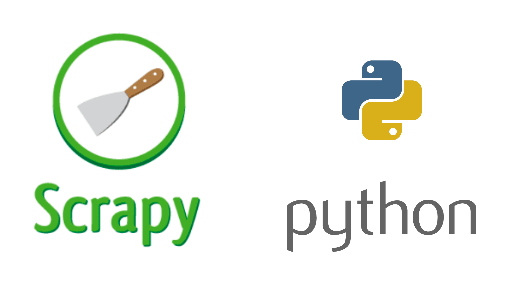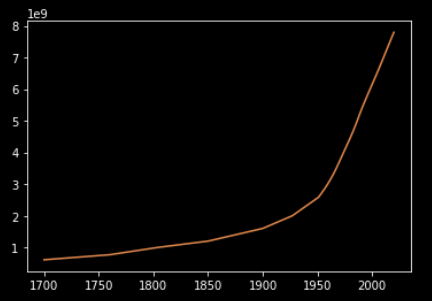# Year-wise population graph using ScrapyI have always been amazed by phenomena like entropy and chaos. At the same time, I feel pleased while seeing straight lines and symmetric curves. So, I love to find patterns in chaos.
Have you heard about the Bell curve? Almost every natural phenomena if studied a long time follows the Bell curve. I thought, why not plot the world population vs year graph, and see how much it matches. We accomplish this by scraping the Worldometer website. To do this, we’ll use the Python framework `scrapy`. Then we’ll use Python’s plotting library to plot that curve. Let’s begin!

## 1. Scraping #

I’ll assume that you already have `scrapy` installed on your machine. We’ll begin by creating a project which I’ll name `popscrape`.

``````\$ scrapy startproject popscrape
``````

To keep things simple, I’ll use a single spider and no proxies. So to create the spider, we’ll put a file named `main.py` at `popscrape/popscrape/spider`.

``````import scrapy

class mainSpider(scrapy.Spider):
name = 'main'
start_urls = [
'https://www.worldometers.info/world-population/world-population-by-year/'
]

def parse(self, response):
raw = response.css('td::text')
i = 0
for _ in range(int(len(raw)/7)):
yield {
'year': raw[i+0].get(),
'population': raw[i+1].get(),
}
i += 7
``````

The code should be pretty straightforward if you’re used to `scrapy`, but it can be little intimidating for newbie readers so I’ll try to explain it. The code initializes a spider named `main` which crawls URLs from the list `start_urls`. The receieved response is then parsed using `parse()`. The list `raw` stores the raw data from the table on the website. The following loop extracts data from `raw` and yields year and population data. To store the crawled data, we run following command in the project’s root directory.

``````\$ scrapy crawl main -o main.jl
``````

This gives us a JSON file named `main.json`.

## 2. Plotting #

We’ll plot the graph using `matplotlib` for which we’ll create `plotter.py` file at the project’s root directory.

``````import json
import matplotlib.pyplot as plt

with open('main.json', 'r') as f:
year=[]
population=[]
for main in main_dict:
year.append(int(main['year']))
population.append(int((main['population'].replace(',',''))))
year.reverse()
population.reverse()
year = year[18:]
population = population[18:]

plt.plot(year,population)
plt.savefig('plot.pdf')
``````

`plotter.py` reads the `main.json` and then stores the population and year data in lists. I dropped the first few elements to make the graph clearer. The data is thus plotted on a graph and saved as `plot.pdf`.

## Conclusion #That’s what we wanted to see! Thus the plot we got can be considered as the starting part of the bell curve. So we can safely assume that we are going to last at least a few more centuries. That concludes this post.
To practice more, I suggest trying to draw a similar graph for month-wise cases of COVID-19. Feel free to comment if you have any doubts.
Happy coding.